# Percent Timer Setting Calculations

Older pivots only have a percent timer. Use this form to calculate a timer setting to apply a particular depth. For partial pivots use the numbers for a 360 degree, full rotation. Use the full rotation calculator to find the approximate amount of time it will take for a pivot to make a full rotation. Calculate the time for a 1" application.

## Percent Setting By Depth

Lane Spacing:
%
Depth of water applied at above setting:
Desired new depth to apply:
New percent timer setting:
%

## Percent Setting by Desired Completion Time

Percent timer setting at known completion time
%
Known completion time:
New desired completion time:
New percent timer setting for new completion time:
%

## Percent Setting By 1" Application

Time for Full Rotation at 100%:
Time for 1" Application:
Application Required:
Percent Timer Setting:
%

### The Equations

These calculators use these formulas to determine the percent timer setting to achieve a specified application depth or completion time.

#### Percent Setting By Depth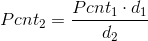Where: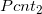= The new pivot percent timer setting to achieve desired depth (as a %)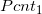= The pivot percent timer setting to achieve known application depth (as a %)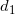= Known application depth (in)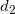= Desired new application depth (in)

#### Percent Setting By Desired Completion Time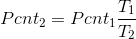Where:= The new pivot percent timer setting to achieve desired completion time (as a %)= The pivot percent timer setting to at known completion time (as a %)= Known completion time (hrs)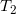= Desired completion time (hrs)

#### Percent Setting By 1&quot Application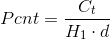Where: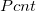= The new pivot percent setting to achieve desired depth (as a %)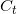= Pivot full rotation time at 100% (hrs)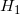= Pivot full rotation time to apply 1 inch (hrs)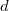= Desired application depth (in)

Reference: Washington State University